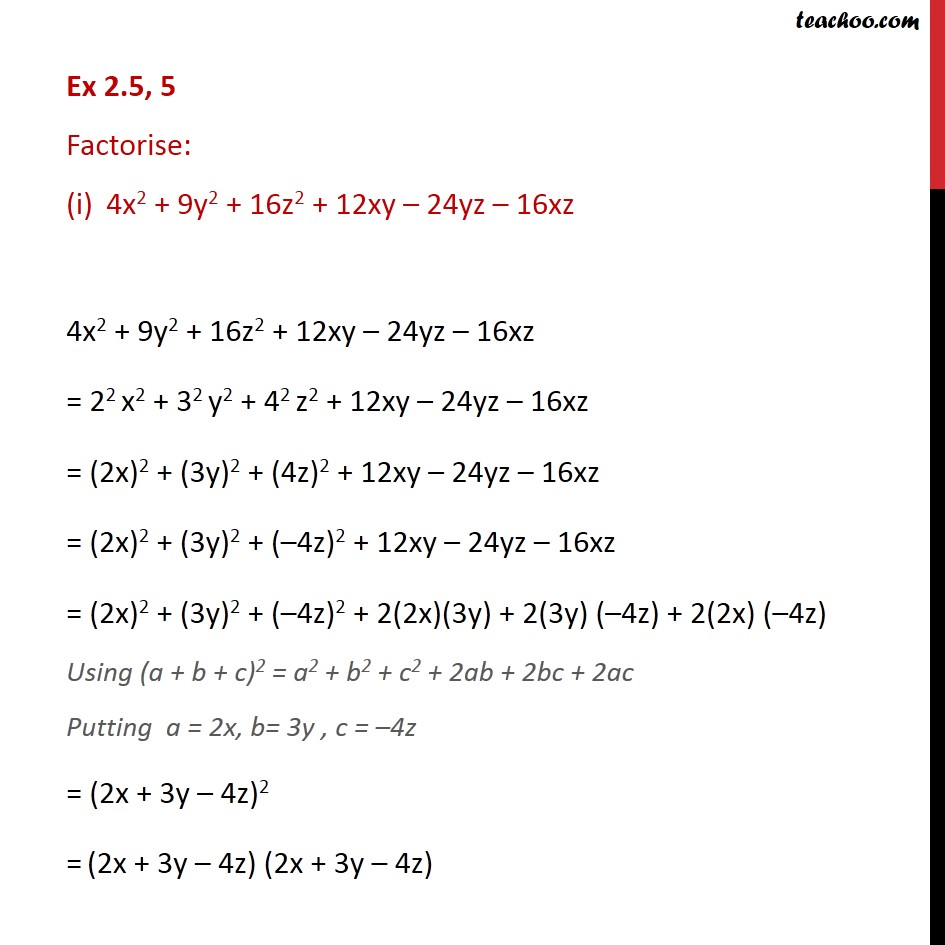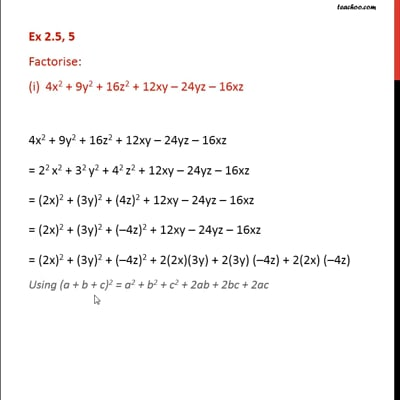Ex 2.5

Chapter 2 Class 9 Polynomials
Serial order wiseThis video is only available for Teachoo black users

Solve all your doubts with Teachoo Black (new monthly pack available now!)

### Transcript

Ex 2.5, 5 Factorise: 4x2 + 9y2 + 16z2 + 12xy – 24yz – 16xz 4x2 + 9y2 + 16z2 + 12xy – 24yz – 16xz = 22 x2 + 32 y2 + 42 z2 + 12xy – 24yz – 16xz = (2x)2 + (3y)2 + (4z)2 + 12xy – 24yz – 16xz = (2x)2 + (3y)2 + (–4z)2 + 12xy – 24yz – 16xz = (2x)2 + (3y)2 + (–4z)2 + 2(2x)(3y) + 2(3y) (–4z) + 2(2x) (–4z) Using (a + b + c)2 = a2 + b2 + c2 + 2ab + 2bc + 2ac Putting a = 2x, b= 3y , c = –4z = (2x + 3y – 4z)2 = (2x + 3y – 4z) (2x + 3y – 4z)